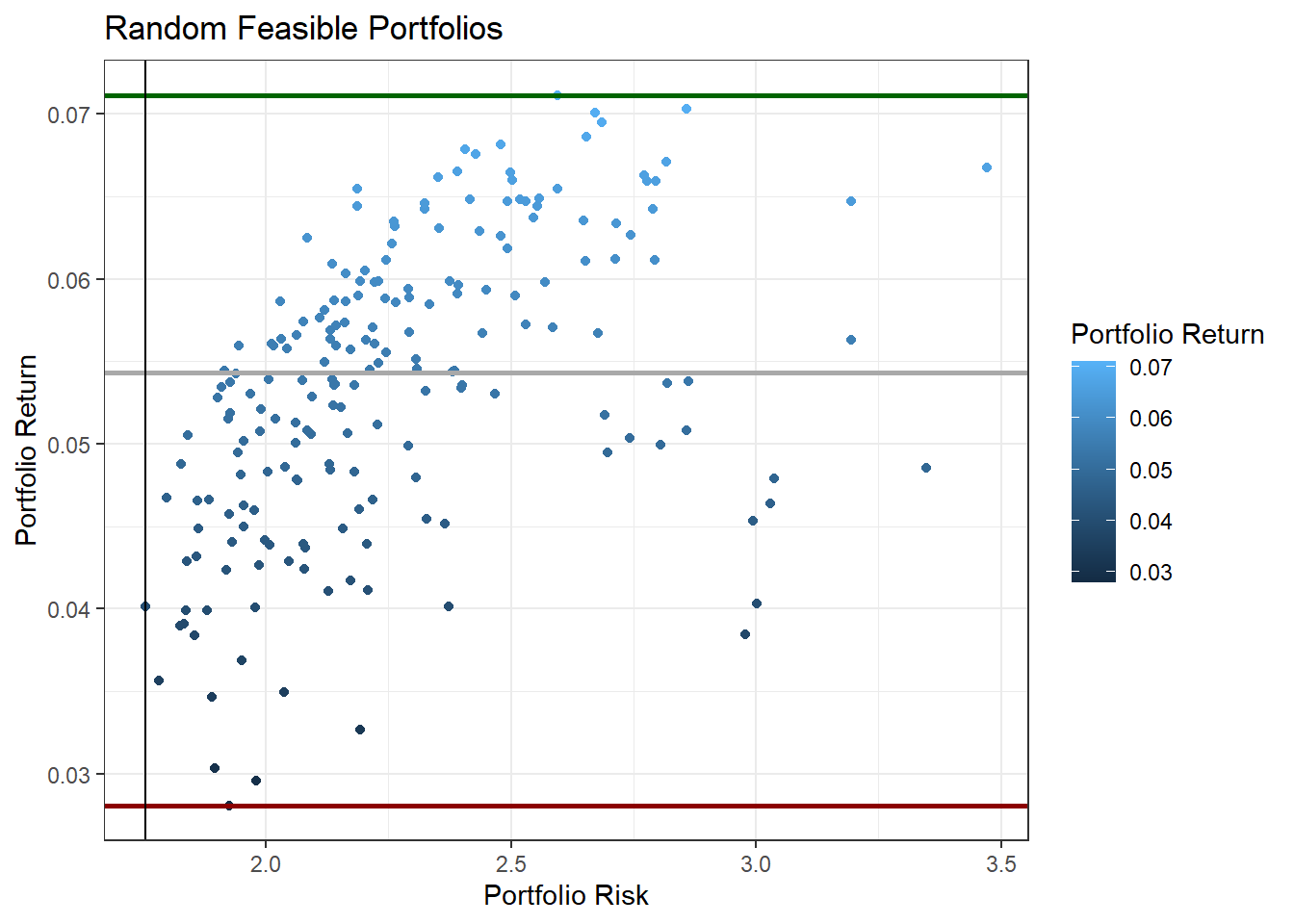## 12.5 Using R to Construct Multi-Asset Portfolio

• This section will contruct a five (5) stock portfolio using prices downloaded from Yahoo finance
• The exercise first creates random weight portfolios then uses two packages PortfolioAnalytics and fPortfolio for risk-return efficient portfolios.

### 12.5.1 Data

• Download 2 year daily prices for BHP.AX, ANZ.AX, WOW.AX, TLS.AX and CSL.AX
• Stocks are chosen from different sectors
library(quantmod)
library(TTR)
# create a vector of stocks
s1 = c("BHP.AX", "ANZ.AX", "WOW.AX", "TLS.AX", "CSL.AX")

data1 = lapply(s1, FUN = function(x) {
ROC(Ad(getSymbols(x, from = "2019-07-01", to = "2021-06-30", auto.assign = FALSE)),
type = "discrete") * 100
})  #%returns

# convert to data frame
ret1 = as.data.frame(do.call(merge, data1))
# change columna names
# remove the first row of missing values
ret1 = ret1[-1, ]
ret1 = data.frame(Date = as.Date(row.names(ret1)), ret1)
row.names(ret1) = NULL
# save the dataframe (not necessarily required, only for
# reproducibility)
saveRDS(ret1, file = "data/port_ret.Rds")
• Plot the data
library(pander)
library(ggplot2)
library(tidyr)
library(ggthemes)
# overview
pander(head(ret1), split.table = Inf)
Date BHP ANZ WOW TLS CSL
2019-07-02 0.8637 -1.45 -0.2726 -0.5222 0.6696
2019-07-03 0.09515 -0.287 2.703 1.312 -0.3486
2019-07-04 -0.5466 1.331 0.7096 0 1.781
2019-07-05 -1.338 -0.03551 0.734 0.5181 1.596
2019-07-08 -1.768 -0.9591 -1.137 -0.7732 -1.491
2019-07-09 1.159 -0.6815 0.6486 0.7792 -0.5874
# convert to long
ret_long = pivot_longer(ret1, cols = -c(Date), values_to = "Return", names_to = "Stock")
# plot

port_p1 = ggplot(ret_long, aes(Date, Return, color = Stock)) + geom_path(stat = "identity") +
facet_grid(Stock ~ .) + theme_minimal() + labs(x = "Date", y = "Returns")
port_p1  #covid crisis period is evident in the plotFigure 12.2: Simple returns

### 12.5.2 Portfolios with random weights

• Calculate the mean returns
• Calculate variance-covariance matrix
• Create series of random weights
• Create series of rerturns and risks
• Create plot
np1 = 200  #number of portfolios
ret2 = ret1[, -1]  #excluding dates
mu1 = colMeans(ret2)  #mean returns
na1 = ncol(ret2)  #number of assets
varc1 = cov(ret2)

riskp1 = NULL  #vector to store risk
retp1 = NULL  #vector to store returns
# using loops here (not aiming for efficiency but demonstration)

for (i in 1:np1) {
w = diff(c(0, sort(runif(na1 - 1)), 1))  # random weights
r1 = t(w) %*% mu1  #matrix multiplication
sd1 = t(w) %*% varc1 %*% w
retp1 = rbind(retp1, r1)
riskp1 = rbind(riskp1, sd1)
}

# create a data frame of risk and return
d_p1 = data.frame(Ret = retp1, Risk = riskp1)
# simple plot
plot(d_p1$Risk, d_p1$Ret, xlab = "Risk", ylab = "Return", main = "Frontier Portfolios",
col = "blue")Figure 12.3: Random Portfolios

• Use ggplot2
library(ggplot2)
# first layer
p1 = ggplot(d_p1, aes(Risk, Ret, colour = Ret))
# scatter plot
p1 = p1 + geom_point()
# scatter plot with density and identified port risk return (highest
# lowest returns and min risk)
p1 + geom_point() + geom_hline(yintercept = c(max(d_p1$Ret), median(d_p1$Ret),
min(d_p1$Ret)), colour = c("darkgreen", "darkgray", "darkred"), size = 1) + geom_vline(xintercept = d_p1[(d_p1$Risk == min(d_p1\$Risk)), ][, 2]) +
labs(colour = "Portfolio Return", x = "Portfolio Risk", y = "Portfolio Return",
title = "Random Feasible Portfolios") + theme_bw()Figure 12.4: Random Portfolios